# Understanding variable scopes in JavaScriptA variable is a named container used for storing values. A piece of information that we might reference multiple times can be stored in a variable for later use or modification. In JavaScript,the value contained inside a variable can be any JavaScript data type, including a number, string,or object.

A variable is a named container used for storing values. A piece of information that we might reference multiple times can be stored in a variable for later use or modification. In JavaScript,the value contained inside a variable can be any JavaScript data type, including a number, string,or object.

In JavaScript, there are two types of scopes Global Scope – Scope outside the outermost function attached to window.Local Scope – Inside the function being executed. Let’s look at the code below. We have a global variable defined in first line in global scope. Then we have a local variable defined inside the function fun().

``````let globalLet = "This is a global variable";

function fun() {
let localLet = "This is a local variable";

console.log(globalLet); // This is a global variable
console.log(localLet); // This is a local variable
}
fun();
``````

Output: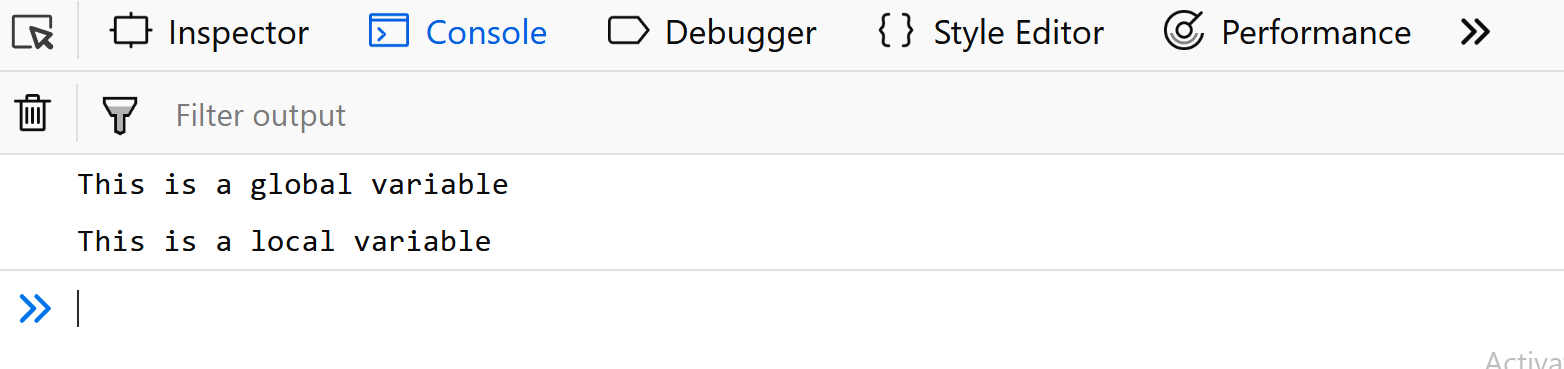When we execute the function fun(), the output shows that both global, as well as local variables, are accessible inside the function as we are able to console.log them. This shows that inside the function we have access to both global variables (declared outside the function) and local variables (declared inside the function). Let’s move the console.log statements outside the function and put them just after calling the function.

``````let globalLet = "This is a global variable";

function fun() {
let localLet = "This is a local variable";

}
fun();
console.log(globalLet); // This is a global variable
console.log(localLet); // localLet is not defined
``````

Output: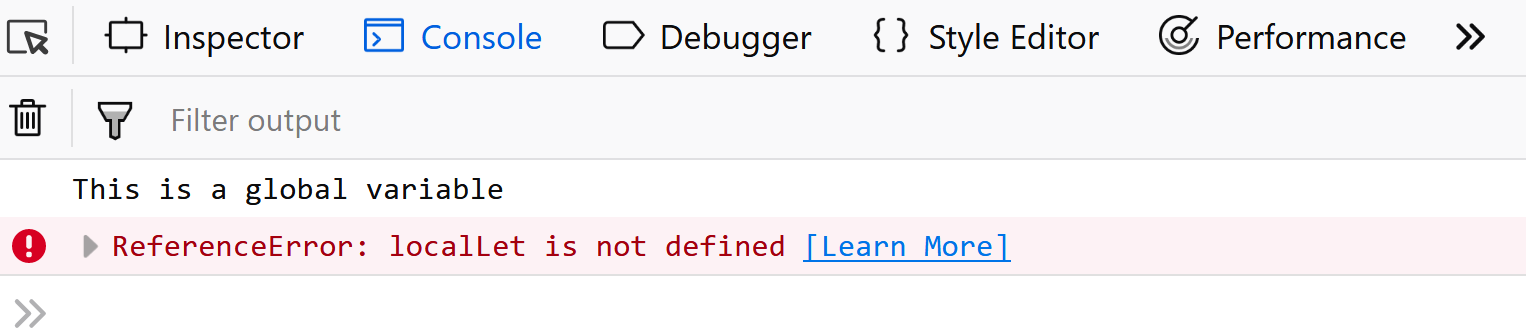We are still able to see the value of the global variable, but for local variable console.log throws an error. This is because now the console.log statements are present in global scope where they have access to global variables but cannot access the local variables.

Word of caution: Whenever you are declaring variables, always use the prefix let. If you don’t use the let keyword, then the variables are by default created in the global scope. For instance, in the above example, let’s just remove the keyword let before the declaration of localLet.

``````let globalLet = "This is a global variable";

function fun() {
localLet = "This is a local variable";
}

fun();
console.log(globalLet); // This is a global variable
console.log(localLet); // This is a local variable
``````

Output:We are now able to console.log the local variable as well because the localLet was created in the global scope as we missed the keyword let while declaring it. What really happened is that as we didn’t use the let keyword, JavaScript first searched the localLet in local scope, then in the global scope. As there was no existing global variable by that name, so it created a new global variable.

One of the most asked questions in interviews is the scenario where the global as well as local variable has the same name. Let’s see what happens then.

``````let globalLet = "This is a global variable";

function fun() {
let globalLet = "This is a local variable";
}
fun();
console.log(globalLet); // This is a global variable
``````

Output: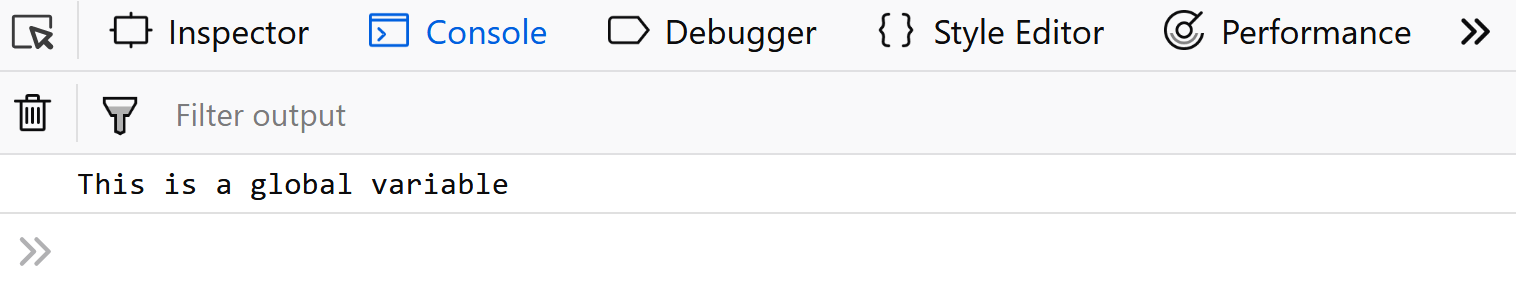In this example, we have the declared a local as well as global variable “globalLet”. What matters here is the scope in which we are accessing it. In the above example, we are accessing it in global scope, so it will output the global variable as local variable is not present in its scope.Let’s move the console.log statement inside the function fun().

``````let globalLet = "This is a global variable";

function fun() {
let globalLet = "This is a local variable";
console.log(globalLet); // This is a local variable
}
fun();
``````

Output: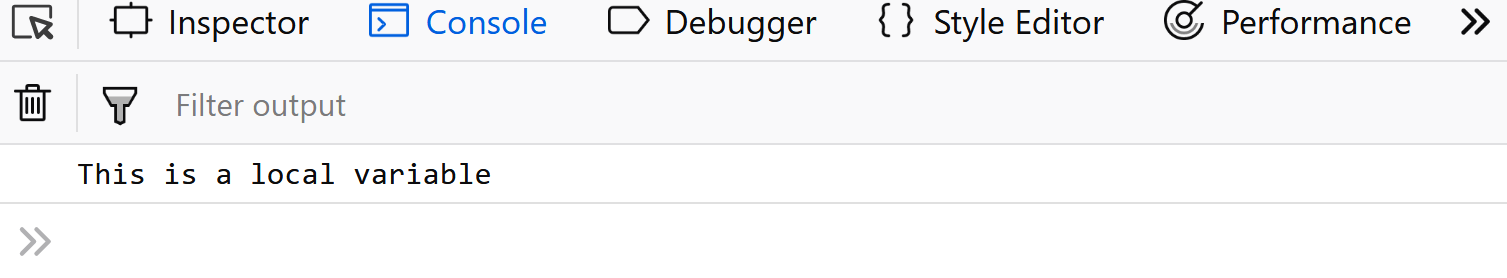Inside the function fun(), both the local as well as global variables are accessible. But when we console.log the variable globalLet, firstly JavaScript tries to find a local variable in the current scope. It finds the local variable and outputs it. Otherwise it would have search for the variable “globalLet” in the outer scope (which in this case is global scope).

What if we want to access the global variable instead of local one here. Well, the window object comes to our rescue. All the global variables are attached to window object and thus we can access the global variable name as shown in example below.

``````let globalLet = "This is a global variable";

function fun() {
let globalLet = "This is a local variable";
console.log(window.globalLet); // This is a global variable
}
fun();
``````

Output:After discussing about scopes in JavaScript, guessing the output of below code fragments should be a cakewalk.

``````function fun(){
function fun2(){
i = 100;
}
fun2();
console.log(i); // 100
}
fun();
``````

Output: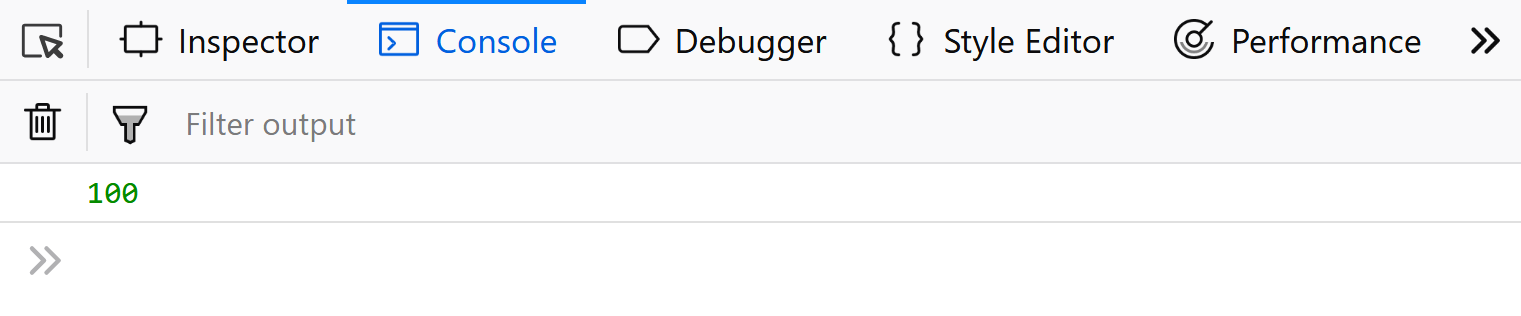``````function fun(){
function fun2(){
let i = 100;
}
fun2();
console.log(i); // i is not defined
}
fun();
``````

Output: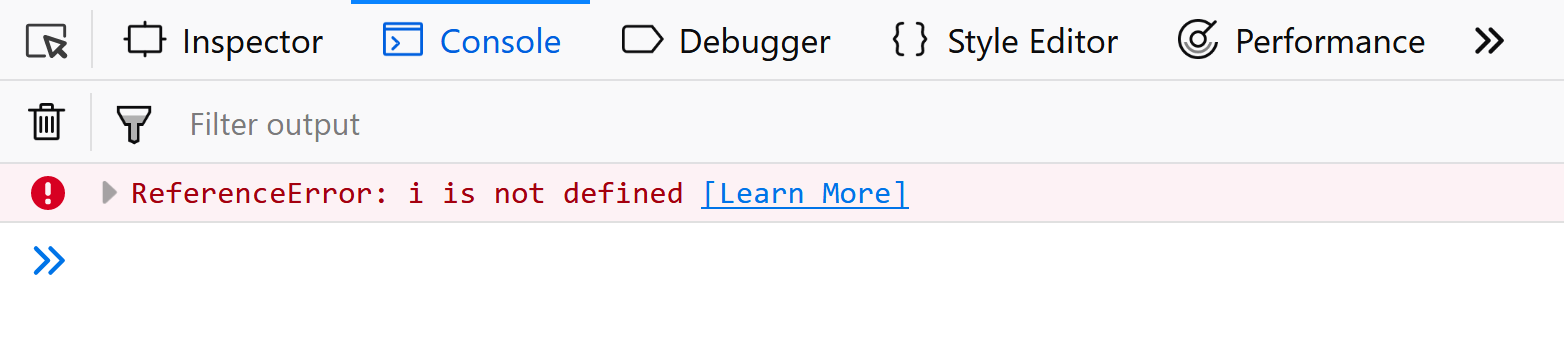In the first example as we didn’t use the keyword let, the variable “i” was assumed to be declared in global scope and thus the output was 100. In the second example, “i” became a local variable and thus was not accessible outside the scope of that function.

Let’s look at another example-

``````function fun(){
if(true){
let i = 100;
}
console.log(i); // i is not defined
}
fun();
``````

Output:After ES2015, we started using let instead of var for declaring variables and also now the if block is also counted as a block scope, hence in the above example we get an error instead of the value 100. If we change the let to var we will get 100 as output as if block was not considered a block scope earlier, only functions were.

## HTML entity encoder decoder Online

With the rapid development in technology, the old ways to do business have changed completely. A lot more advanced and developed ways are ...